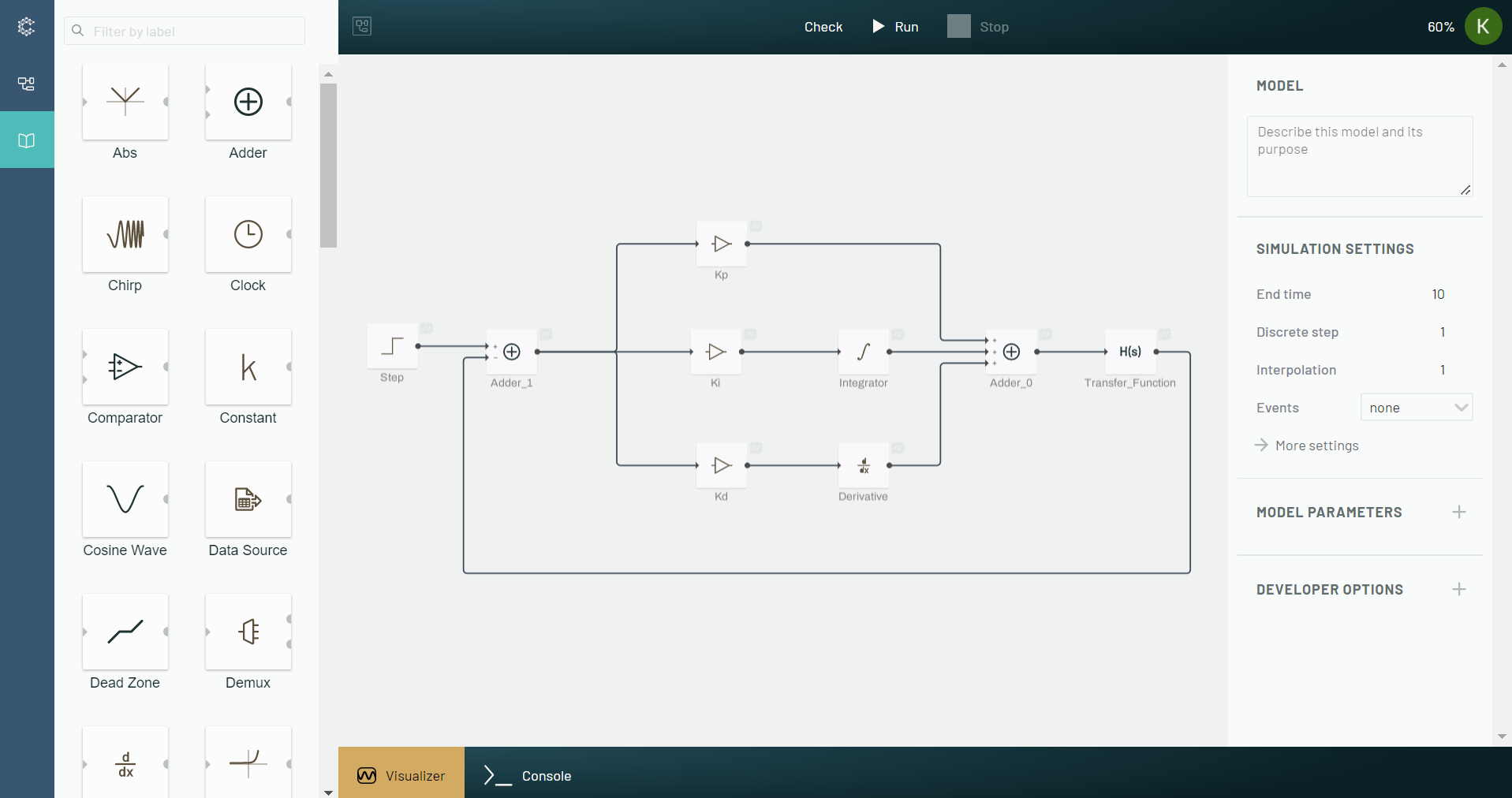March 25, 2022

# Block diagram importance in Systems DesignThere are multiple ways to represent systems and engineers will typically use the one that most easily addresses the issue that they have. The ability to program using block diagram graphical user interfaces is important, especially in control systems and signals processing. However, even though Python is becoming ubiquitous in the development world, there are very few open-source tools that let users shift from Python’s text based development UI to a Python graphical UI. This is evidenced by the numerous engineers who have inquired as to why this functionality does not exist and what options they have available. For example, a quick Google search for “Simulink for Python” “MATLAB Simulink alternative” “How to run python code on Simulink” or “Open source Simulink” each yields millions of results.

In this post, we will focus on two representations: difference equations, and block diagrams.

## Difference equations

$$y[n] = x[n] -x[n-1]$$

This representation is preferred for numerical scientific computing because it is mathematically concise and precise. For example, let’s assume $$x$$ was a unit sample response where every value is $$0$$ except at $$n = 0$$ where it is $$1$$. Given $$x[n] = 𝛿 [n]$$, you can define all the possibilities of $$y$$:

$$y[-1] = x[-1] - x[-2] = 0 - 0 = 0$$

$$y = x - x[-1] = 1 - 0 = 1$$

$$y = x - x = 0 - 1 = -1$$

$$y = x - x = 0 - 0 = 0$$

And at all other values of $$n$$, $$x = 0$$ and $$y = 0$$. Conversely, you could also determine all the possibilities of $$x$$ given $$y$$. Its strength is that it is declarative because it informs the user of the rules the system obeys. However, its weakness is that it isn’t necessarily clear what you can do with the system.

## Block diagrams

This block diagram representation is mathematically equivalent to the difference equation $$y[n] = x[n] - x[n-1]$$ aboveHowever, it has three main strengths:

First, it has more information because the arrows indicate the signal flow path. An engineer can quickly see that $$x$$ is the input and $$y$$ is the output of the system so there is no confusion around cause and effect.

Second, it is easier to code. Engineers do not have to learn a specific language syntax. Instead, they can visually put blocks together and connect them using arrows. The code generated from the visual blocks would be equivalent to writing the code in a text based UI (e.g., using Jupyter Notebooks for Python).

Third, it shows how to build the system in hardware. Reviewing this block diagram, an engineer would immediately know that they would need to have a signal source with two paths, one that directly goes to the adder, and the second that is inverted and delayed.

Hopefully, the benefits of block diagram representations of systems are clear by now.

At Collimator, our goal is to build engineering software that can be used for both model-based system engineering (MSBE) and model-based design (MBD) and allows engineers to code in the way that they are most comfortable. With Collimator, engineers can use a block diagram interface when modeling hybrid dynamical systems that require a more visual and imperative approach. They can also build their own custom function blocks using open source libraries such as Pandas, NumPy, Scipy, TensorFlow and Matplotlib, or write their own Python code when a more computational and declarative approach is desired.